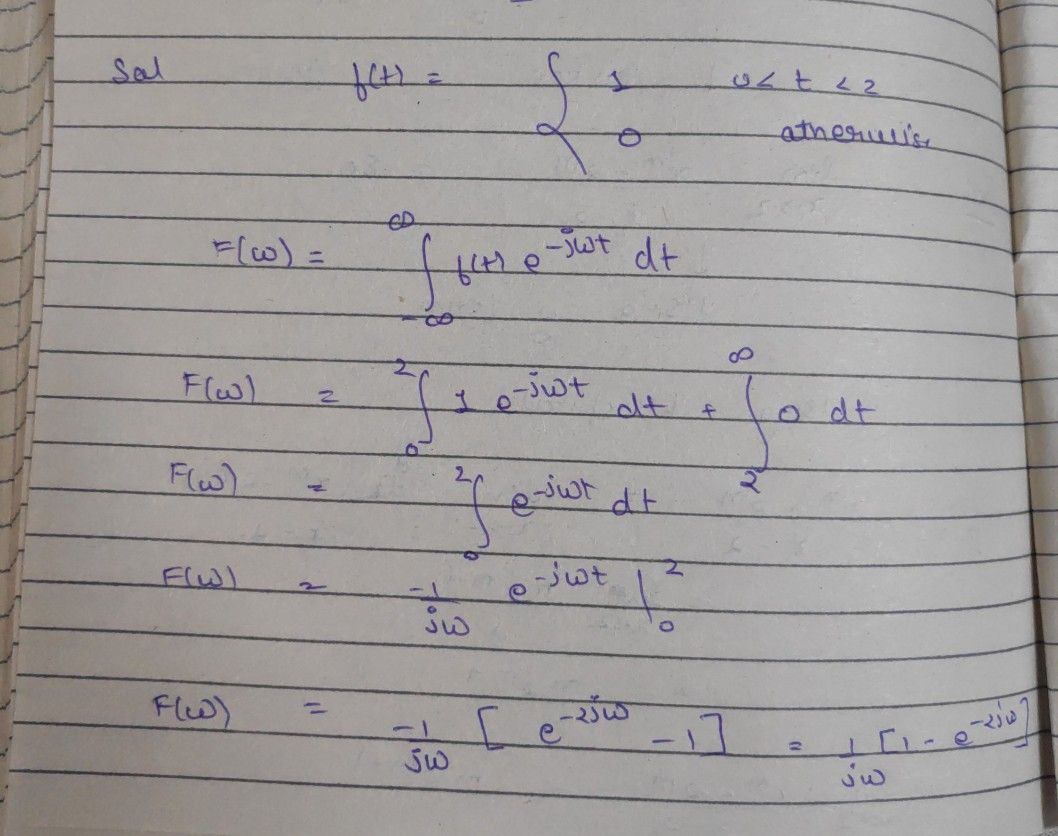Symbol
ProblemProblem $21$ Find the Fourier transform for the following functions, $a\right)$ $f\left(t\right)= \begin{cases} 1 \\ 0 \end{cases}$ $0<t<2$ otherwise $110$ $Man|\times 5$
Calculus
Search count: 107
SolutionQanda teacher - nrohitTell me if you find any proble